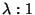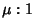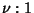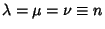## Routh's Theorem

If the sides of a Triangle are divided in the ratios,, and, the Cevians form a central Triangle whose Area is(1)

whereis the Area of the original Triangle. For,(2)

For, 2, 3, ..., the areas are 0, 1/7, 4/13, 3/7, 16/31, 25/43, .... The Area of the Triangle formed by connecting the division points on each side is(3)

Routh's theorem gives Ceva's Theorem and Menelaus' Theorem as special cases.